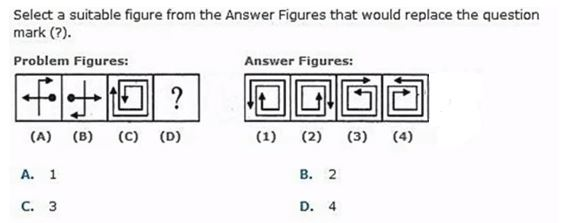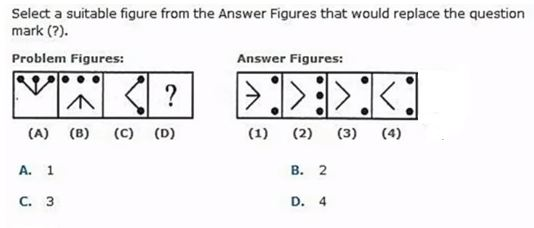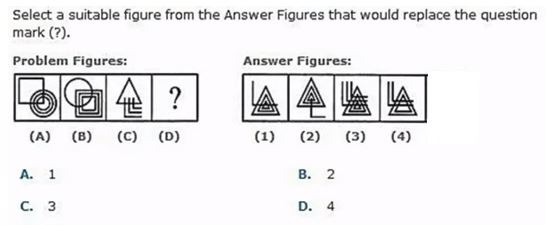# Picture Pattern Analogies

#### Video Lesson on Picture Pattern Analogies

ANALOGY means correspondence or similarities between two figures. And we have to find the similarity between the two figures to get our answer.

### Rotation of FigureIn this type of question, we have to find similarity in the rotation of figure. Two figures are related to each other through a specific rotation of the same figure.

Like in the above question figure (A) is rotated 180 degrees to obtain figure (B).

Now we have to apply the same logic to the next two figures to obtain the answer figure.

As we can see when we rotated the figure (C) by 180 degrees we get the figure shown in figure (2) of answer figures given.

The Answer is B

### Dislocation of FigureIn these types of questions, we have to see how the first figure is getting dislocated or is getting combined to get our answer figure.

Like in the above question figure (A) is 3 dots connected with 3 straight lines but in figure (B) the dots are getting dislocated from the lines and lines get inserted.

We have to apply the same logic to get our answer figure.

So in our answer figure, the dots and lines must need to be disjointed from each other and line gets inverted. So figure (C) follows this pattern hence our answer will be C.

### Interchange of ImagesIn these types of questions, images get interchanged from one another to get the other image. Like in the above question, in figure (A) circle is at the corner of the square and in figure (B) circle takes the position of the square and vice versa.

So we have to apply the same logic to get our answer figure.

Figure (C) is the combination of triangle and three line.

So in our answer figure, the position of lines and triangle must be interchanged. This can be seen in figure (1) ANS- (A)